Tree Traversals

1. Write the inorder traversal of the tree.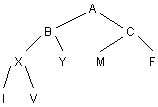2. Write the postorder traversal of this tree.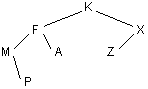3. Given the binary tree, write the preorder traversal.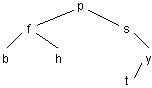4. Write the tree in postfix notation. Evaluate.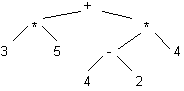5. Write an inorder traversal of this expression tree.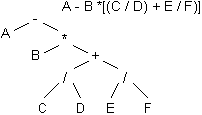6. Draw the tree for which the inorder output is:
X A Y B Z C D Q E

7. Draw the binary tree containing n nodes whose preorder sequence is the same as its inorder sequence.

8. Draw the binary tree containing n nodes whose inorder sequence is the same as its postorder sequence.

9. A list of integers is read in, one at a time, and an ordered binary tree is constructed. Next the tree is traversed and the integers are printed. Which traversal would result in a printout which duplicates the original order of the list of integers?
A. preorder
B. postorder
C. inorder
D. none of these

Continue to:  Unit 8 / Prev / Next